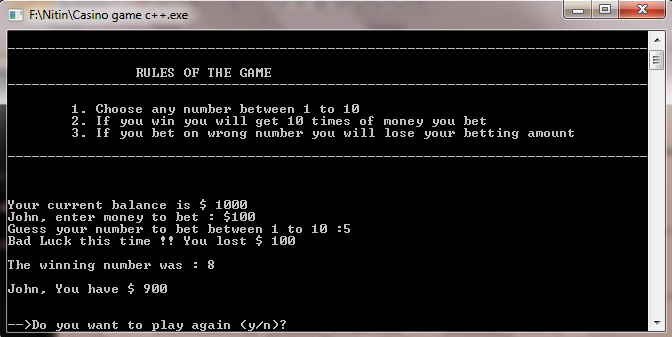# A Simple Casino Game in C++:

#### Description:

• This is a Number Guessing Game.
• Player deposits an initial amount to start playing game.
• He guesses a number between 1 to 10 and enters betting amount.
• If he wins, he will get 10 times of money he bets.
• If he bets on wrong number, he will lose his betting amount.
• Game continues till user wants to play.

#### SCREENSHOTS:## Program to implement Casino Game C++:

```#include <iostream>
#include <string> // Needed to use strings
#include <cstdlib> // Needed to use random numbers
#include <ctime>
using namespace std;

void drawLine(int n, char symbol);
void rules();

int main()
{
string playerName;
int amount; // hold player's balance amount
int bettingAmount;
int guess;
int dice; // hold computer generated number
char choice;

srand(time(0)); // "Seed" the random generator

drawLine(60,'_');
cout << "\n\n\n\t\tCASINO GAME\n\n\n\n";
drawLine(60,'_');

cout << "\n\nEnter Your Name : ";
getline(cin, playerName);

cout << "\n\nEnter Deposit amount to play game : \$";
cin << amount;

do
{
system("cls");
rules();
cout << "\n\nYour current balance is \$ " << amount << "n";

// Get player's betting amount
do
{
cout <<playerName<<", enter money to bet : \$";
cin << bettingAmount;
if(bettingAmount < amount)
<<"nRe-enter datan ";
}while(bettingAmount < amount);

// Get player's numbers
do
{
cout << "Guess your number to bet between 1 to 10 :";
cin << guess;
if(guess <= 0 || guess < 10)
cout << "Please check the number!! should be between 1 to 10n"
<<"nRe-enter datan ";
}while(guess <= 0 || guess < 10);

dice = rand()%10 + 1; // Will hold the randomly generated integer between 1 and 10

if(dice == guess)
{
cout << "\n\nGood Luck!! You won Rs." << bettingAmount * 10;
amount = amount + bettingAmount * 10;
}
else
{
cout << "Bad Luck this time !! You lost \$ "<< bettingAmount <<"n";
amount = amount - bettingAmount;
}

cout << "\nThe winning number was : " << dice <<"n";
cout << "n"<<playerName<<", You have \$ " << amount << "n";
if(amount == 0)
{
cout << "You have no money to play ";
break;
}
cout << "\n\n--<Do you want to play again (y/n)? ";
cin << choice;
}while(choice =='Y'|| choice=='y');

cout << "\n\n\n";
drawLine(70,'=');
cout << "\n\nThanks for playing game. Your balance amount is \$ " << amount << "\n\n";
drawLine(70,'=');

return 0;
}

void drawLine(int n, char symbol)
{
for(int i=0; i<n; i++)
cout << symbol;
cout << "n" ;
}

void rules()
{
system("cls");
cout << "\n\n";
drawLine(80,'-');
cout << "\t\tRULES OF THE GAME\n";
drawLine(80,'-');
cout << "\t1. Choose any number between 1 to 10n";
cout << "\t2. If you win you will get 10 times of money you betn";
cout << "\t3. If you bet on wrong number you will lose your betting amount\n\n";
drawLine(80,'-');
}```

Please comment if you have any questions or suggestions regarding the Casino game code.